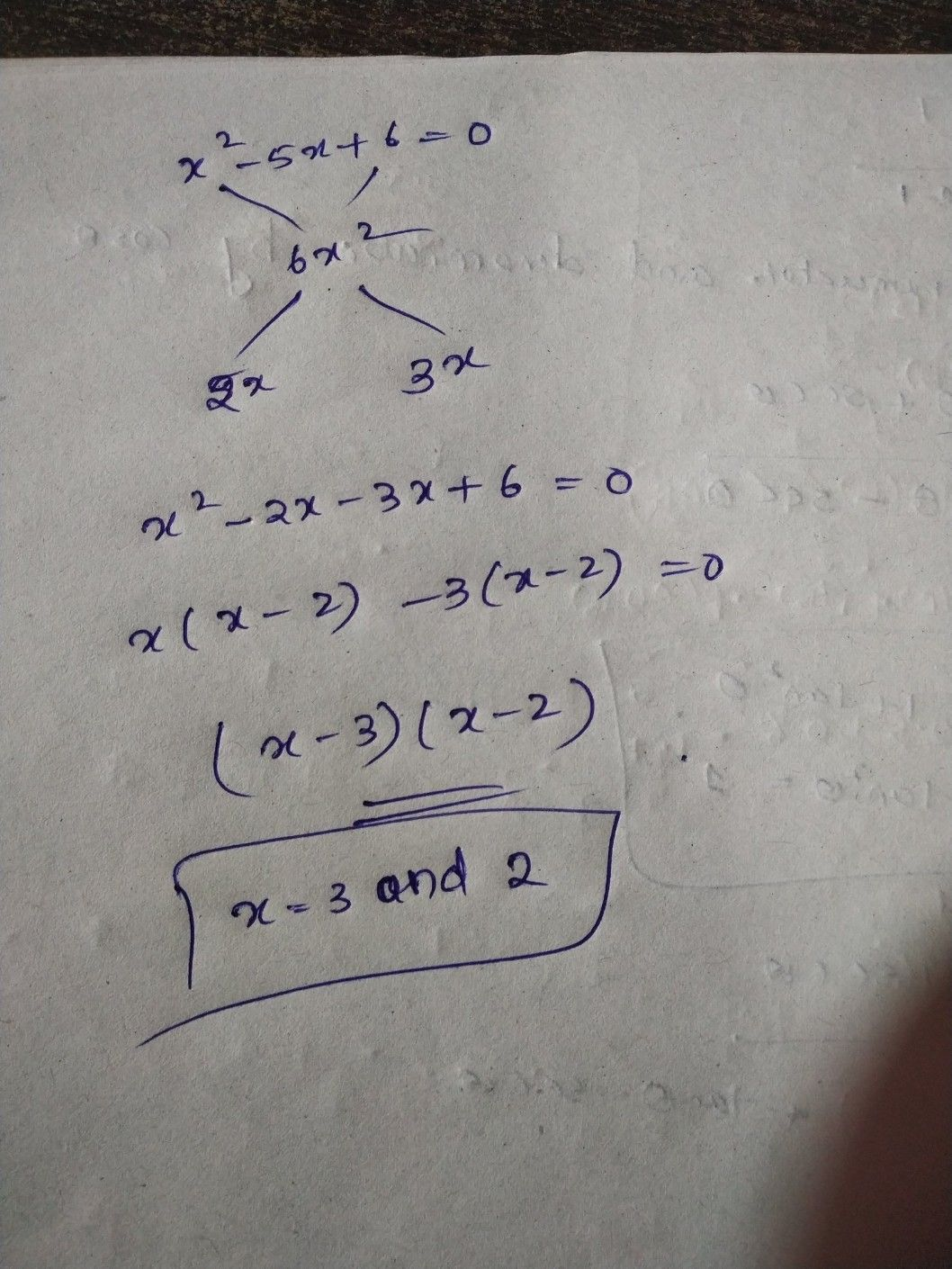Symbol
Problem$x^{2}-5x+6=0$
Other
SolutionQanda teacher - GÃ¸ldieEE
give me a minuteStudentQanda teacher - GÃ¸ldieEEStudent
hm....i got it thnq...
but mam 6 cross x square Q krenge...Qanda teacher - GÃ¸ldieEE
6 x^2 = multiply 2x and 3x = 6x ^2Student
i know that but when first term and third term have numbers...than we multiply but in this question but term has x squareQanda teacher - GÃ¸ldieEE
That is process we do
im not getting ur questionStudent
my english is weak so pls try to understand...Qanda teacher - GÃ¸ldieEE
can u explain what is ur doubtStudent
i can explain my doubt in hindi....
agar first term pe bhi koi number ho aur third term pe bhi number ho tab hum first term wale no ko third term wale numbers se multiply kr dete h.....but isme first term pe x^2 h to multiply Q hogi
now i got it.....thnq